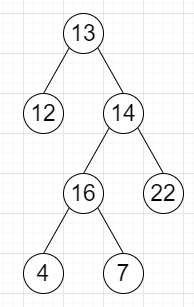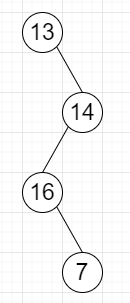# Program to delete all leaves with even values from a binary tree in Python

Suppose we have we have a binary tree, we will repeatedly delete all leaves that have even values. After deleting all, if it has only root with even values, that will be deleted also.

So, if the input is likethen the output will beTo solve this, we will follow these steps −

• Define a function solve() . This will take root

• if root is null, then

• return null

• left of root := solve(left of root)

• right of root := solve(right of root)

• if root is leaf and data of root is even, then

• return null

• return root

Let us see the following implementation to get better understanding −

## Example

class TreeNode:
def __init__(self, data, left = None, right = None):
self.data = data
self.left = left
self.right = right

def inorder(root):
if root:
inorder(root.left)
print(root.data, end = ', ')
inorder(root.right)

class Solution:
def solve(self, root):
if not root:
return None

root.left = self.solve(root.left)
root.right = self.solve(root.right)

if not root.left and not root.right and root.data % 2 == 0:
return None
return root

ob = Solution()
root = TreeNode(13)
root.left = TreeNode(12)
root.right = TreeNode(14)
root.right.left = TreeNode(16)
root.right.right = TreeNode(22)
root.right.left.left = TreeNode(4)
root.right.left.right = TreeNode(7)
ob.solve(root)
inorder(root)

## Input

root = TreeNode(13)
root.left = TreeNode(12)
root.right = TreeNode(14)
root.right.left = TreeNode(16)
root.right.right = TreeNode(22)
root.right.left.left = TreeNode(4)
root.right.left.right = TreeNode(7)

## Output

13, 16, 7, 14,